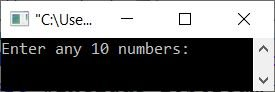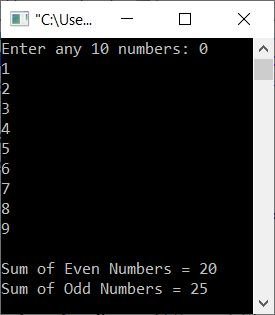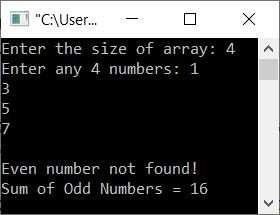# C++ program to find the sum of even and odd numbers

This article provides some programs in C++ that find and print the sum of all even and odd numbers from a list of some random numbers entered by the user. The program is created in the following two ways:

## Using a for loop, find the sum of even and odd numbers

The question is, "Write a C++ program that receives 10 numbers from the user and prints the sum of all even and all odd numbers." The program given below is its answer:

```#include<iostream>

using namespace std;
int main()
{
int arr, i, eve=0, odd=0;
cout<<"Enter any 10 numbers: ";
for(i=0; i<10; i++)
cin>>arr[i];
for(i=0; i<10; i++)
{
if(arr[i]%2==0)
eve = eve+arr[i];
else
odd = odd+arr[i];
}
cout<<"\nSum of Even Numbers = "<<eve;
cout<<"\nSum of Odd Numbers = "<<odd;
cout<<endl;
return 0;
}```

This snapshot shows the initial output produced by the above C++ program on finding the sum of even and odd numbers from a given array:Now supply the input, say 0, 1, 2, 3, 4, 5, 6, 7, 8, 9 as ten numbers for the array, and then press the ENTER key to find and print the sum of even and odd numbers from all these given 10 numbers, as shown in the snapshot given below:#### Modified Version of the Previous Program

This is a modified version of the previous program that allows the user to specify the array's size as well as the array's elements, or numbers. Also, this program included some messages to print when no even or odd number was found in the given list of numbers.

```#include<iostream>

using namespace std;
int main()
{
int n, i, eve=0, odd=0;
cout<<"Enter the size of array: ";
cin>>n;
int arr[n];
cout<<"Enter any "<<n<<" numbers: ";
for(i=0; i<n; i++)
{
cin>>arr[i];
if(arr[i]%2==0)
eve += arr[i];
else
odd += arr[i];
}
if(eve==0)
else
cout<<"\nSum of Even Numbers = "<<eve;
if(odd==0)
else
cout<<"\nSum of Odd Numbers = "<<odd;
cout<<endl;
return 0;
}```

Here is its sample run with user input of 4 as size and 1, 3, 5, 7 as four numbers:In the above program, the statement is:

`eve += arr[i];`

is the same as

`eve = eve + arr[i];`

## Using a while loop, find the sum of even and odd numbers

This is the last program of this article, created using the while loop. Because the while loop lacks initialization and update statements. Therefore, we need to do initialization before the loop and an update statement in the body of the loop, as shown in the program given here:

```#include<iostream>

using namespace std;
int main()
{
int n, i=0, eve=0, odd=0;
cout<<"Enter the size of array: ";
cin>>n;
int arr[n];
cout<<"Enter any "<<n<<" numbers: ";
while(i<n)
{
cin>>arr[i];
if(arr[i]%2==0)
eve += arr[i];
else
odd += arr[i];
i++;
}
if(eve==0)
else
cout<<"\nSum of Even Numbers = "<<eve;
if(odd==0)
else
cout<<"\nSum of Odd Numbers = "<<odd;
cout<<endl;
return 0;
}```

This program produces the same output as the previous program.

C++ Quiz

« Previous Program Next Program »